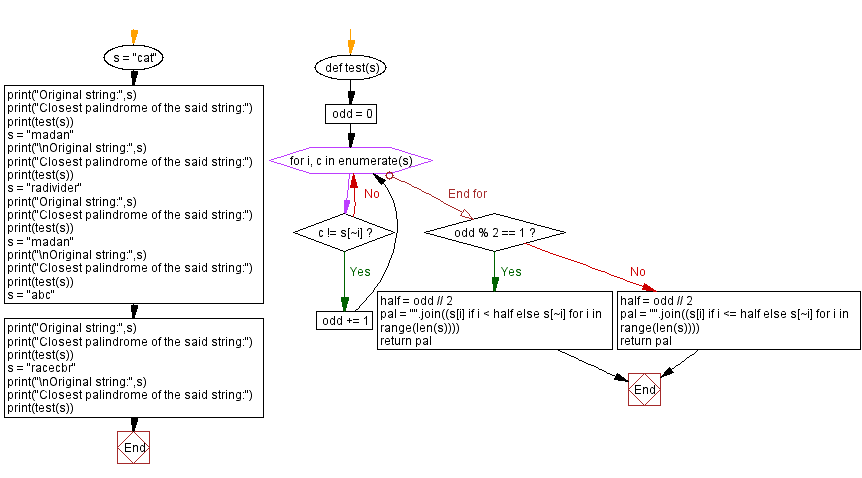﻿ Python: Find the closest palindrome - w3resource# Python: Find the closest palindrome

## Python Programming Puzzles: Exercise-93 with Solution

Write a Python program to find the closest palindrome from a given string.

```Input:
cat
Output:
cac

Input:
Output:
Input:
Output:

Input:
Output:
Input:
abc
Output:
aba

Input:
racecbr
Output:
racecar
```

Sample Solution:

Python Code:

``````#License: https://bit.ly/3oLErEI

def test(s):
odd = 0
for i, c in enumerate(s):
if c != s[~i]:
odd += 1
if odd % 2 == 1:
half = odd // 2
pal = "".join((s[i] if i < half else s[~i] for i in range(len(s))))
return pal
else:
half = odd // 2
pal = "".join((s[i] if i <= half else s[~i] for i in range(len(s))))
return pal

s = "cat"
print("Original string:",s)
print("Closest palindrome of the said string:")
print(test(s))
print("\nOriginal string:",s)
print("Closest palindrome of the said string:")
print(test(s))
print("Original string:",s)
print("Closest palindrome of the said string:")
print(test(s))
print("\nOriginal string:",s)
print("Closest palindrome of the said string:")
print(test(s))
s = "abc"
print("Original string:",s)
print("Closest palindrome of the said string:")
print(test(s))
s = "racecbr"
print("\nOriginal string:",s)
print("Closest palindrome of the said string:")
print(test(s))
``````

Sample Output:

```Original string: cat
Closest palindrome of the said string:
cac

Closest palindrome of the said string:
Closest palindrome of the said string:

Closest palindrome of the said string:
Original string: abc
Closest palindrome of the said string:
aba

Original string: racecbr
Closest palindrome of the said string:
racecar
```

Flowchart:## Visualize Python code execution:

The following tool visualize what the computer is doing step-by-step as it executes the said program:

Python Code Editor :

Have another way to solve this solution? Contribute your code (and comments) through Disqus.

What is the difficulty level of this exercise?

Test your Programming skills with w3resource's quiz.

﻿

## Python: Tips of the Day

Clamps num within the inclusive range specified by the boundary values x and y:

Example:

```def tips_clamp_num(num,x,y):
return max(min(num, max(x, y)), min(x, y))
print(tips_clamp_num(2, 4, 6))
print(tips_clamp_num(1, -1, -6))
```

Output:

```4
-1
```